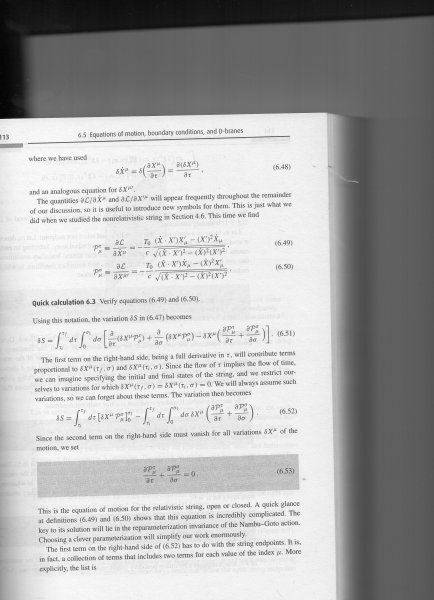# String motion

moriheru
My question concerns motion of relativistic Strings, as the reader with a great a mount of deductive skills can deduce :).The question is: How can I derive the P's. More acuratly speaking why is the numerator how it is.
I am refering to Zwiebach chapter 6.
Thanks for any clarifications and sorry about the zoom.

#### Attachments

Staff Emeritus
2021 Award
Please type your question in, using LaTex if you need to express a formula. That's better than asking us to try and figure out what is in a tiny blurry picture.

Gold Member
Take the Lagrangian you have, and find its derivative...
The Nambu Goto lagrangian he has derived is : $L = - \frac{T_0}{c} \sqrt{ - det \gamma } = - \frac{T_0}{c} \sqrt{ ( \dot{X} X' )^2 - \dot{X}^2 X'^2 }$
Forgeting the prefactor:
$\frac{\partial L}{\partial \dot{X}^\rho} \propto \frac{2 ( \dot{X} X' ) X'_\rho - 2 X'^2 \dot{X}_\rho}{2 \sqrt{ ( \dot{X} X' )^2 - \dot{X}^2 X'^2 } }$
Similarily for the other...
If you have some problems with these derivatives of inner products:
$\frac{\partial }{\partial \dot{X}^\rho} ( \dot{X}^\mu X'_\mu )^2 =\frac{\partial }{\partial \dot{X}^\rho} \dot{X}^\mu X'_\mu \dot{X}^\nu X'_\nu = \delta_{\rho}^{\mu} X'_\mu \dot{X}^\nu X'_\nu + \dot{X}^\mu X'_\mu \delta_{\rho}^{\nu} X'_\nu = X'_\rho \dot{X}^\nu X'_\nu + \dot{X}^\mu X'_\mu X'_\rho =2 (\dot{X}^\nu X'_\nu ) X'_\rho$

The only thing you use is the orthonormality relation $\frac{\partial x^a}{\partial x^b}= \delta_b^a$

Last edited:
•moriheru
moriheru
Take the Lagrangian you have, and find its derivative...
The Nambu Goto lagrangian he has derived is : $L = - \frac{T_0}{c} \sqrt{ - det \gamma } = - \frac{T_0}{c} \sqrt{ ( \dot{X} X' )^2 - \dot{X}^2 X'^2 }$
Forgeting the prefactor:
$\frac{\partial L}{\partial \dot{X}^\rho} \propto \frac{2 ( \dot{X} X' ) X'_\rho - 2 X'^2 \dot{X}_\rho}{2 \sqrt{ ( \dot{X} X' )^2 - \dot{X}^2 X'^2 } }$
Similarily for the other...
If you have some problems with these derivatives of inner products:
$\frac{\partial }{\partial \dot{X}^\rho} ( \dot{X}^\mu X'_\mu )^2 =\frac{\partial }{\partial \dot{X}^\rho} \dot{X}^\mu X'_\mu \dot{X}^\nu X'_\nu = \delta_{\rho}^{\mu} X'_\mu \dot{X}^\nu X'_\nu + \dot{X}^\mu X'_\mu \delta_{\rho}^{\nu} X'_\nu = X'_\rho \dot{X}^\nu X'_\nu + \dot{X}^\mu X'_\mu X'_\rho =2 (\dot{X}^\nu X'_\nu ) X'_\rho$

The only thing you use is the orthonormality relation $\frac{\partial x^a}{\partial x^b}= \delta_b^a$

Thanks! A like is yours.

moriheru
Take the Lagrangian you have, and find its derivative...
The Nambu Goto lagrangian he has derived is : $L = - \frac{T_0}{c} \sqrt{ - det \gamma } = - \frac{T_0}{c} \sqrt{ ( \dot{X} X' )^2 - \dot{X}^2 X'^2 }$
Forgeting the prefactor:
$\frac{\partial L}{\partial \dot{X}^\rho} \propto \frac{2 ( \dot{X} X' ) X'_\rho - 2 X'^2 \dot{X}_\rho}{2 \sqrt{ ( \dot{X} X' )^2 - \dot{X}^2 X'^2 } }$
Similarily for the other...
If you have some problems with these derivatives of inner products:
$\frac{\partial }{\partial \dot{X}^\rho} ( \dot{X}^\mu X'_\mu )^2 =\frac{\partial }{\partial \dot{X}^\rho} \dot{X}^\mu X'_\mu \dot{X}^\nu X'_\nu = \delta_{\rho}^{\mu} X'_\mu \dot{X}^\nu X'_\nu + \dot{X}^\mu X'_\mu \delta_{\rho}^{\nu} X'_\nu = X'_\rho \dot{X}^\nu X'_\nu + \dot{X}^\mu X'_\mu X'_\rho =2 (\dot{X}^\nu X'_\nu ) X'_\rho$

The only thing you use is the orthonormality relation $\frac{\partial x^a}{\partial x^b}= \delta_b^a$

But why would one have a inner product?

Gold Member
What do you mean by why you have inner products? they are there when you wrote:

$\gamma = \begin{pmatrix} \dot{X} \cdot \dot{X} & \dot{X} \cdot X' \\ X' \cdot \dot{X} & X' \cdot X' \end{pmatrix}$
The $\cdot$ denotes inner product, and in this case the Lorentzian product $x \cdot y = \eta_{\mu \nu} x^\mu y^\nu$...
Now why is that the case? because you have already derived the induced metric:
$\gamma_{ab} = \eta_{\mu \nu} \partial_a X^\mu \partial_b X^\nu \equiv \partial_a X \cdot \partial_b X$

Gold Member
Or if you didn't ask exactly that, then remember:
$\frac{d}{dx} f(g(x)) = \frac{d}{dg}f(g) \frac{dg(x)}{dx}$

So the $\sqrt{...}$ will give the $\frac{1}{2\sqrt{...}}$
and you still have to take the derivatives of $(...)$ wrt your initial derivative.

•moriheru
moriheru
Thanks fine from here!